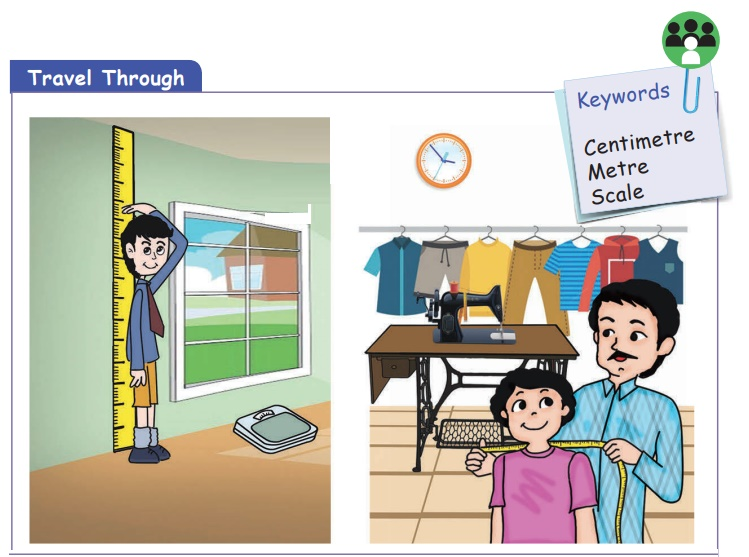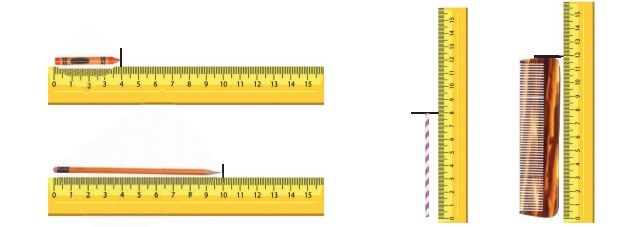Home | | Maths 2nd Std | Standard units of measuring length

# Standard units of measuring length

Teacher’s Note: Teacher can facilitate the children to discuss about the use of standard units of measurement.

Standard units of measuring length

Travel ThroughKeywords: Centimetre, Metre, Scale

Teacher’s Note: Teacher can facilitate the children to discuss about the use of standard units of measurement.

Learn

Measuring by standard unit

Objects of greater length are measured in metre (m) and objects of smaller length are measured in centimetre (cm).Photo frame is measured in Centimetre.Saree is measured in metre.

Practice

Tick () the appropriate unit to measure the following objects.Learn

Measuring length

Let us learn to measure the length of the objects using scale.Teacher’s Note: Teacher should encourge children to count the centimetre. The object can be kept at different value say 3cm or 5cm instead of 0 to ensure the children count and right the length of the object.

Practice

Observe the picture in previous page and write the length of the objects. One is done for you.Length of the comb is 12 cm.

Length of the straw is 10 cm.

Length of the pencil Is 14  cm.

Length of the crayon is 7 cm.

Activity

Fill in the boxes by measuring the length of the objects using handspan, eraser and centimetre scale.Teacher’s Note:

i. Teacher can make the students to measure various objects in the surrounding by guessing and by actual measurement.

ii. Enable the students to appreciate the need for standard tool for measuring length.

Tags : Measurement | Term 1 Chapter 4 | 2nd Maths , 2nd Maths : Term 1 Unit 4 : Measurement
Study Material, Lecturing Notes, Assignment, Reference, Wiki description explanation, brief detail
2nd Maths : Term 1 Unit 4 : Measurement : Standard units of measuring length | Measurement | Term 1 Chapter 4 | 2nd Maths